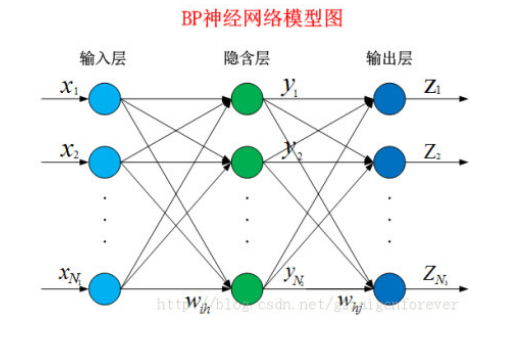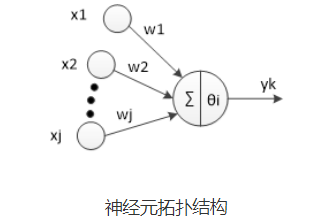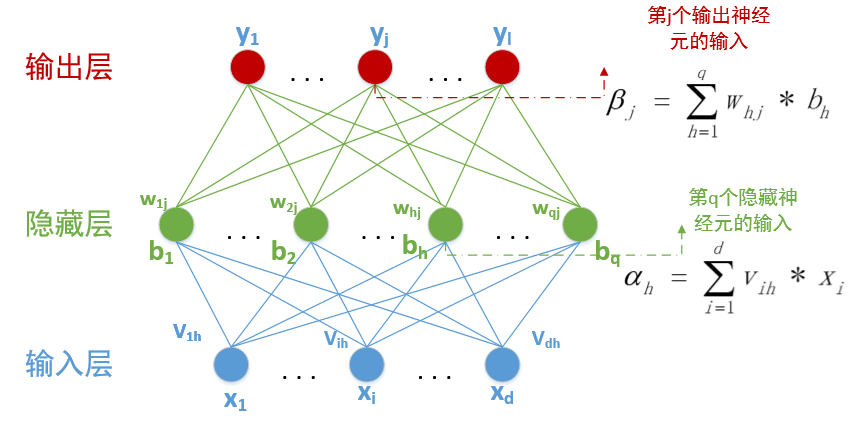# 详解BP神经网络

#BackPropagation Neuron NetWok
BP神经网络学习算法可以说是目前最成功的神经网络学习算法。显示任务中使用神经网络时，大多数是使用BP算法进行训练.
在我看来BP神经网络就是一个”万能的模型+误差修正函数“，每次根据训练得到的结果与预想结果进行误差分析，进而修改权值和阈值，一步一步得到能输出和预想结果一致的模型。举一个例子：比如某厂商生产一种产品，投放到市场之后得到了消费者的反馈，根据消费者的反馈，厂商对产品进一步升级，优化，从而生产出让消费者更满意的产品。这就是BP神经网络的核心
下面就让我们来看看BP算法到底是什么东西。BP网络由输入层、隐藏层、输出层组成。给定训练集***D***={(x1,y1),(x2,y2…(xn,yn)},其中xnϵRd，ynϵRl,表示输入示例由d个属性组成，输出l维实值变量。现在，我们看看如何求得输出值，以及怎么由输出值调整权值和阈值。神经元是以生物研究及大脑的响应机制而建立的拓扑结构网络，模拟神经冲突的过程，多个树突的末端接受外部信号，并传输给神经元处理融合，最后通过轴突将神经传给其它神经元或者效应器。神经元的拓扑结构如图：对于第i个神经元，X1、X2、…、Xj为神经元的输入，输入常为对系统模型关键影响的自变量，W1、W2、…、Wj为连接权值调节各个输入量的占重比。将信号结合输入到神经元有多种方式，选取最便捷的线性加权求和可得neti神经元净输入:
N e t i n = ∑ i = 1 n w i ∗ x i Net_{in}=\sum_{i=1}^{n}{w_i*x_i}
θ \theta i表示该神经元的阈值，根据生物学中的知识，只有当神经元接收到的信息达到阈值是才会被激活。因此，我们将 N e t i n Net_{in} θ j \theta_j 进行比较，然后通过激活函数处理以产生神经元的输出。
激活函数：激活函数这里我们不多重述。如果输出值有一定的范围约束，比如用来分类，一般我们用的最多的是Sigmod函数，它可以把输入从负无穷大到正无穷大的信号变换成0到1之间输出。如果没有约束的话，我们可以使用线性激活函数(即权值相乘之和)。这样我们得到的输出为：
y j = f ( N e t i n − θ j ) y_j=f(Net_{in}-\theta_j)
我们可以将公式化简一下，设第一个输入永远值为 θ \theta ,权值为-1，则我们可以得到公式：
y j = f ( ∑ i = 0 n w i ∗ x i ) y_j=f(\sum_{i=0}^nw_i*x_i)
其中w0=-1,x0= θ \theta j,其中f为选择的激活函数。
已经知道在BP神经网络模型中，我们有三层结构，输入层、隐藏层、输出层,因此输入层到隐藏层的权值，设为 v i h v_{ih} ,隐藏层第h个神经元的阈值我们设为 γ h \gamma_h 。隐藏层到输出层的权值，设为 w h j w_{hj} ,输出层第j个神经元的阈值我们用 θ j \theta_j 表示。在下面这张图里，有d输入神经元,q个隐藏神经元，隐藏有q个隐藏神经元阈值， l l 个输出神经元，因此有 l l 个输出神经元阈值。其中 β j \beta_j 中的 b h = f ( α h − γ h ) b_h=f(\alpha_h-\gamma_h) 。隐藏层和输出层的激活函数，在这里我们暂时全部用 S i g m o d Sigmod 函数。
在某个训练示例 ( x k , y k ) (x_k,y_k) 中，假设神经网络的训练输出为 y k , = ( y 1 k , , y 2 k , , ⋯   , y l k , ) y_{k^,}=({y_1^{k^,},y_2^{k^,},\cdots,y_l^{k^,}}) ,输出为 l l 维向量，其中
y i k , = f ( β i − θ i ) y_i^{k^,}=f(\beta_i-\theta_i)
那么这次预测结果的误差我们可以用最小二乘法表示：
E k = 1 2 ∑ j = 1 l ( y j k , − y j k ) 2 E_k=\frac{1}{2}\sum_{j=1}^l(y_j^{k^,}-y_j^k)^2
而我们现在要做的就是根据这个误差去调整 （ d + l + 1 ） q + l （d+l+1）q+l 个参数的值，一步一步缩小 E k E_k 。那么从现在开始，我们就要进入数学的世界了。这里我们使用最常用的算法：梯度下降法来更新参数。函数永远是沿着梯度的方向变化最快，那么我们对每一个需要调整的参数求偏导数，如果偏导数>0,则要按照偏导数相反的方向变化；如果偏导数<0，则按照此方向变化即可。于是我们使用-1*偏导数则可以得到参数需要变化的值。同时我们设定一个学习速率 η \eta ，这个学习速率不能太快，也不能太慢。太快可能会导致越过最优解；太慢可能会降低算法的效率。(具体设多少就属于玄学调参的领域了)。因此我们可以得到一个参数调整公式：
P a r a m + = − η ∂ E k ∂ P a r a m Param+=-\eta\frac{\partial E_k}{\partial Param}
首先我们看看隐藏层到输出层的权值调整值：
Δ w h j = − η ∂ E k ∂ w h j \Delta w_{hj}=-\eta\frac{\partial E_k}{\partial w_{hj}}
好，我们从上到下缕一缕这个偏导该怎么求，我们把每一个公式都罗列出来：
#####  1.输入层到隐藏层：
α h = ∑ i = 1 d v i h ∗ x i ⋯ ⋯ ⋯ ⋯ ⋯ ⋯ ⋯ ( 1 ) \alpha_h=\sum_{i=1}^dv_{ih}*x_i \cdots \cdots \cdots \cdots \cdots \cdots \cdots(1)
∣ x 1 x 2 x 3 ⋯ x d ∣ ⋅ ∣ v 11 v 12 v 13 ⋯ v 1 q v 21 v 22 v 23 ⋯ w 2 q ⋅ ⋅ ⋅ ⋅ ⋅ ⋅ ⋅ ⋅ ⋅ ⋅ ⋅ ⋅ ⋅ ⋅ ⋅ v d 1 w d 2 w d 3 ⋯ w d q ∣ \begin{vmatrix} x_1 &x_2 &x_3&\cdots& x_d \end{vmatrix} \cdot \begin{vmatrix} v_{11} &v_{12} &v_{13}& \cdots& v_{1q} \\ v_{21} &v_{22} &v_{23}& \cdots& w_{2q} \\ \cdot & \cdot &\cdot & \cdot &\cdot \\ \cdot & \cdot &\cdot & \cdot &\cdot \\ \cdot & \cdot &\cdot & \cdot &\cdot \\ v_{d1} &w_{d2}&w_{d3} &\cdots & w_{dq} \end{vmatrix}
#####  2.经过隐藏层的激活函数：
b h = f ( α h − γ h ) ⋯ ⋯ ⋯ ⋯ ⋯ ⋯ ⋯ ( 2 ) b_h=f(\alpha_h-\gamma_h) \cdots \cdots \cdots \cdots \cdots \cdots \cdots(2)
#####  3.隐藏层到输出层：
β j = ∑ h = 1 q w h j ∗ b h ⋯ ⋯ ⋯ ⋯ ⋯ ⋯ ⋯ ( 3 ) \beta_j=\sum_{h=1}^qw_{hj}*b_h \cdots\cdots \cdots \cdots \cdots \cdots \cdots(3)
#####  用矩阵表示
∣ b 1 b 2 b 3 ⋯ b q ∣ ⋅ ∣ w 11 w 12 w 13 ⋯ w 1 l w 21 w 22 w 23 ⋯ w 2 l ⋅ ⋅ ⋅ ⋅ ⋅ ⋅ ⋅ ⋅ ⋅ ⋅ ⋅ ⋅ ⋅ ⋅ ⋅ w q 1 w q 2 w q 3 ⋯ w q l ∣ \begin{vmatrix} b_1 &b_2 &b_3&\cdots& b_q \end{vmatrix} \cdot \begin{vmatrix} w_{11} &w_{12} &w_{13}& \cdots& w_{1l} \\ w_{21} &w_{22} &w_{23}& \cdots& w_{2l} \\ \cdot & \cdot &\cdot & \cdot &\cdot \\ \cdot & \cdot &\cdot & \cdot &\cdot \\ \cdot & \cdot &\cdot & \cdot &\cdot \\ w_{q1} &w_{q2}&w_{q3} &\cdots & w_{ql} \end{vmatrix}

#####  4.经过输出层的激活函数：
y j k , = f ( β j − θ j ) ⋯ ⋯ ⋯ ⋯ ⋯ ⋯ ⋯ ( 4 ) ) y_j^{k^,}=f(\beta_j-\theta_j)\cdots \cdots \cdots \cdots \cdots \cdots \cdots(4))
#####  5.误差：
E k = 1 2 ∑ j = 1 l ( y j k , − y j k ) 2 ⋯ ⋯ ⋯ ⋯ ⋯ ⋯ ⋯ ( 5 ) E_k=\frac{1}{2}\sum_{j=1}^l(y_j^{k^,}-y_j^k)^2 \cdots \cdots \cdots \cdots \cdots \cdots \cdots(5)
综上我们可以得知 w h j w_{hj} 先影响 β j \beta_j ,再影响 y j k , y_j^{k^,} ,最后影响 E k E_k ,(一个 w w 权值只会影响一个 β \beta )所以我们可得：
Δ w h j = − η ∂ E k ∂ w h j = − η ∂ E k ∂ y j k , ⋅ ∂ y j k , ∂ β j ⋅ ∂ β j ∂ w h j ⋯ ( 6 ) \Delta w_{hj}=-\eta\frac{\partial E_k}{\partial w_{hj}}=-\eta\frac{\partial E_k}{\partial y_j^{k^,}}\cdot \frac{\partial y_j^{k^,}}{\partial \beta_j} \cdot\frac{\partial \beta_j}{\partial w_{hj}}\cdots(6)

g j = ∂ E k ∂ y j k , ⋅ ∂ y j k , ∂ β j = ( y j k , − y j k ) ⋅ f ’ ( β j − θ j ) ⋯ ( 7 ) g_j=\frac{\partial E_k}{\partial y_j^{k^,}}\cdot \frac{\partial y_j^{k^,}}{\partial \beta_j}=(y_j^{k^,}-y_j^k)\cdot f^{’}(\beta_j-\theta_j) \cdots(7)
而我们选择的激活函数是 S i g m o d Sigmod 函数，该函数具有一个很好的性质
f ( x ) = 1 1 + e − x ⋯ f ′ ( x ) = f ( x ) ( 1 − f ( x ) ) ⋯ ( 8 ) f(x)=\frac{1}{1+e^{-x}}\cdots f^{'}(x)=f(x)(1-f(x)) \cdots(8)
所以我们有：
f ′ ( β j − θ j ) = f ( β j − θ j ) ⋅ ( 1 − f ( β j − θ j ) ) = y j k ′ ⋅ ( 1 − y j k ′ ) ⋯ ( 9 ) f^{'}(\beta_j-\theta_j)=f(\beta_j-\theta_j)\cdot (1-f(\beta_j-\theta_j))=y_j^{k^{'}}\cdot (1-y_j^{k^{'}}) \cdots(9)
综合 f o r m u l a ( 6 ) ( 7 ) ( 9 ) formula(6)(7)(9) 我们可得：
Δ w h j = − η ∂ E k ∂ w h j = − η g i b h = − η ( y j k ′ − y j k ) ⋅ y j k ′ ⋅ ( 1 − y j k ′ ) ⋅ b h ⋯ ( 10 ) \Delta w_{hj}=-\eta\frac{\partial E_k}{\partial w_{hj}}=-\eta g_i b_h=-\eta (y_j^{k^{'}}-y_j^k) \cdot y_j^{k^{'}}\cdot (1-y_j^{k^{'}})\cdot b_h \cdots(10)
####  同理：
Δ θ j = − η ∂ E k ∂ θ j = − η ∂ E k ∂ y j k ′ ⋅ ∂ y j k ′ ∂ θ j = η ⋅ g j ⋯ ( 11 ) \Delta \theta_j=-\eta \frac{\partial E_k}{\partial \theta_j}=-\eta \frac{\partial E_k}{\partial y_j^{k^{'}}}\cdot\frac{\partial y_j^{k^{'}}}{\partial \theta_j}=\eta\cdot g_j \cdots(11)
我们再看看 Δ v i h \Delta v_{ih} 的值怎么求，还是由 f o r m u l a ( 1 ) , ( 2 ) , ( 3 ) , ( 4 ) , ( 5 ) formula(1),(2),(3),(4),(5) 推导，一个 v v 权值会影响所有的 β \beta
Δ v i h = − η e h x i ⋯ ⋯ ⋯ ⋯ ( 12 ) \Delta v_{ih}=-\eta e_h x_i \cdots\cdots\cdots\cdots(12)
Δ γ h = η e h ⋯ ⋯   ⋯ ( 13 ) \Delta \gamma_h=\eta e_h \cdots \cdots\ \cdots(13)
其中
e h = （ ∑ j = 1 l ∂ E k ∂ β j ⋅ ∂ β j ∂ b j ） ⋅ f ′ ( α h − γ h ) = ( ∑ j = 1 l ( y j k , − y j k ) ⋅ f ’ ( β j − θ j ) ⋅ w h j ) ⋅ f ′ ( α h − γ h ) ⋯ ⋯ ⋯ ( 14 ) e_h=（\sum_{j=1}^l \frac{\partial E_k}{\partial \beta_j}\cdot \frac{\partial \beta_j}{\partial b_j}）\cdot f^{'}(\alpha_h-\gamma_h)=(\sum_{j=1}^l(y_j^{k^,}-y_j^k)\cdot f^{’}(\beta_j-\theta_j) \cdot w_{hj})\cdot f^{'}(\alpha_h-\gamma_h) \cdots \cdots \cdots(14)
####  至此，我们所有得公式都推导完毕了，剩下做的就是设定一个迭代终止条件，可以是误差小于一定值时终止递归，也可以是设定迭代次数。这样一个BP神经网络模型就算是设计结束。
java实现代码和实验数据在我的github上面

01-10
11-309万+03-254760
10-1118万+
07-28695
06-083万+
05-02
09-011万+
04-114万+
07-272万+